Courses

# MCQ : Circles - 3

## 10 Questions MCQ Test Mathematics (Maths) Class 10 | MCQ : Circles - 3

Description
This mock test of MCQ : Circles - 3 for Class 10 helps you for every Class 10 entrance exam. This contains 10 Multiple Choice Questions for Class 10 MCQ : Circles - 3 (mcq) to study with solutions a complete question bank. The solved questions answers in this MCQ : Circles - 3 quiz give you a good mix of easy questions and tough questions. Class 10 students definitely take this MCQ : Circles - 3 exercise for a better result in the exam. You can find other MCQ : Circles - 3 extra questions, long questions & short questions for Class 10 on EduRev as well by searching above.
QUESTION: 1

Solution:
QUESTION: 2

### A circle touches x-axis at A and y-axis at B. If O is origin and OA = 5 units, then diameter of the circle is

Solution:

OA = OB ⇒ OB = 5
AC = BC [Radii]
⇒ OACB is a square.
⇒ AC = OA = 5
⇒ Diameter =10 units.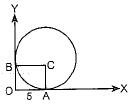QUESTION: 3

### At point A on a diameter AB of a circle of radius 10 cm, tangent XAY is drawn to the circle. The length of the chord CD parallel to XY at a distance 16 cm from A is

Solution: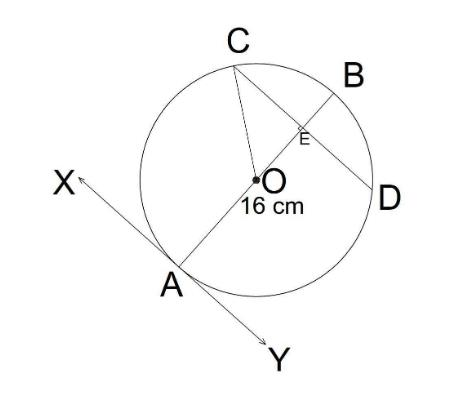In the given figure

XY is the tangent to the circle

We know that tangent is at right angle with the line joining the tangent point to the centre of the circle

Therefore,

∠OAY = 90°

∵ CD is parallel to XY

Therefore,

∠OAY = ∠BED = 90° (Corresponding angles)

Again,

Given that

AE = 16 cm

And the radius of the circle is 10 cm

Therefore,

AO = 10 cm

∴ OE = AE - AO = 16 - 10 = 6 cm

Also,

OC = 10 cm (Radius of the circle)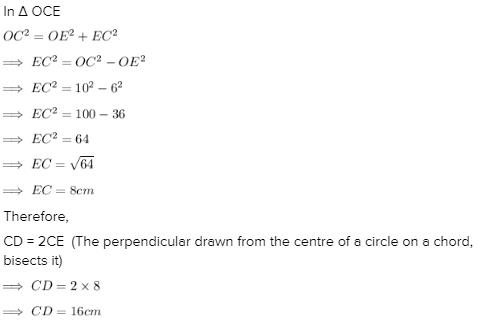QUESTION: 4

From a point P which is at a distance of 13 cm from the centre O of a circle of radius 5 cm, the pair of tangents PQ and PR to the circle are drawn. Then the area of the quadrilateral PQOR is

Solution: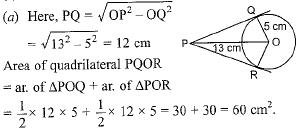QUESTION: 5

Two circles touch each other externally at C and AB is common tangent of circles, then ∠ACB is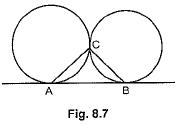Solution:
QUESTION: 6

In figure AT is a tangent to the circle with centre O such that OT = 4 cm and ∠OTA = 30°. Then AT is equal to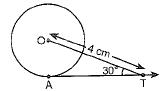Solution: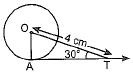∠OAT = 90° [∵ Tangent and radius are ⊥ to each other at the point of contact
In right-angle ΔOAT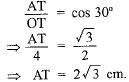QUESTION: 7

In Fig. 8.8, if PA anti PB are tangents to the circle with centre O such that ∠APB = 50°, then ∠OAB is equal to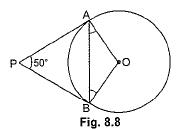Solution:
QUESTION: 8

In figure if O is centre of a circle, PQ is a chord and the tangent PR at P makes an angle of 50° with PQ, then ∠POQ is equal to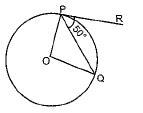Solution:

OP ⊥ PR [∵ Tangent and radius are ⊥ to each other at the point of contact]
∠OPQ = 90° - 50° = 40°
OP - OQ [Radii]
∴ ∠OPQ = ∠OQP = 40°
In ΔOPQ,
⇒ ∠POQ + ∠OPQ + ∠OQP = 180°
⇒ ∠POQ + 40° + 40° = 180°
∠POQ = 180° - 80° = 100°.

QUESTION: 9

If ΔABC is circumscribing a circle in the Fig. 8.12. The length of AB is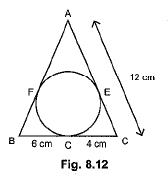Solution:
QUESTION: 10

From a point X, the length of the tangent to a circle is 20 cm and the distance of X from the centre is 25 cm. The radius of the circle is

Solution: